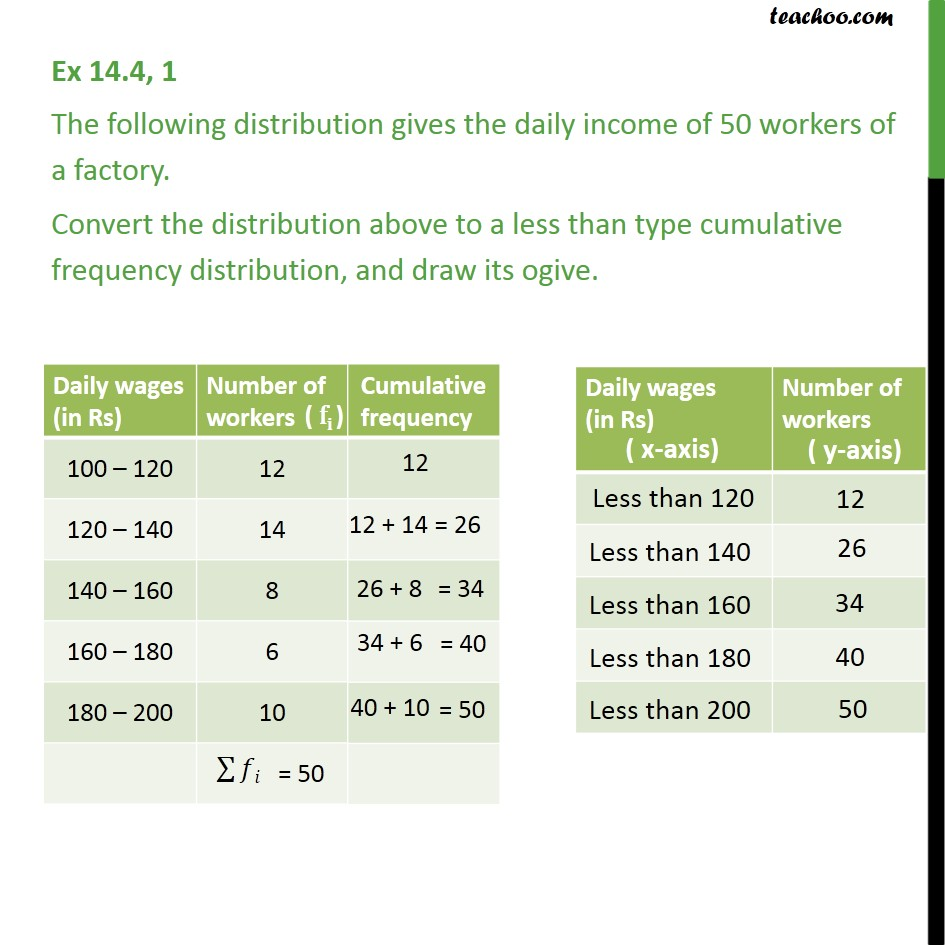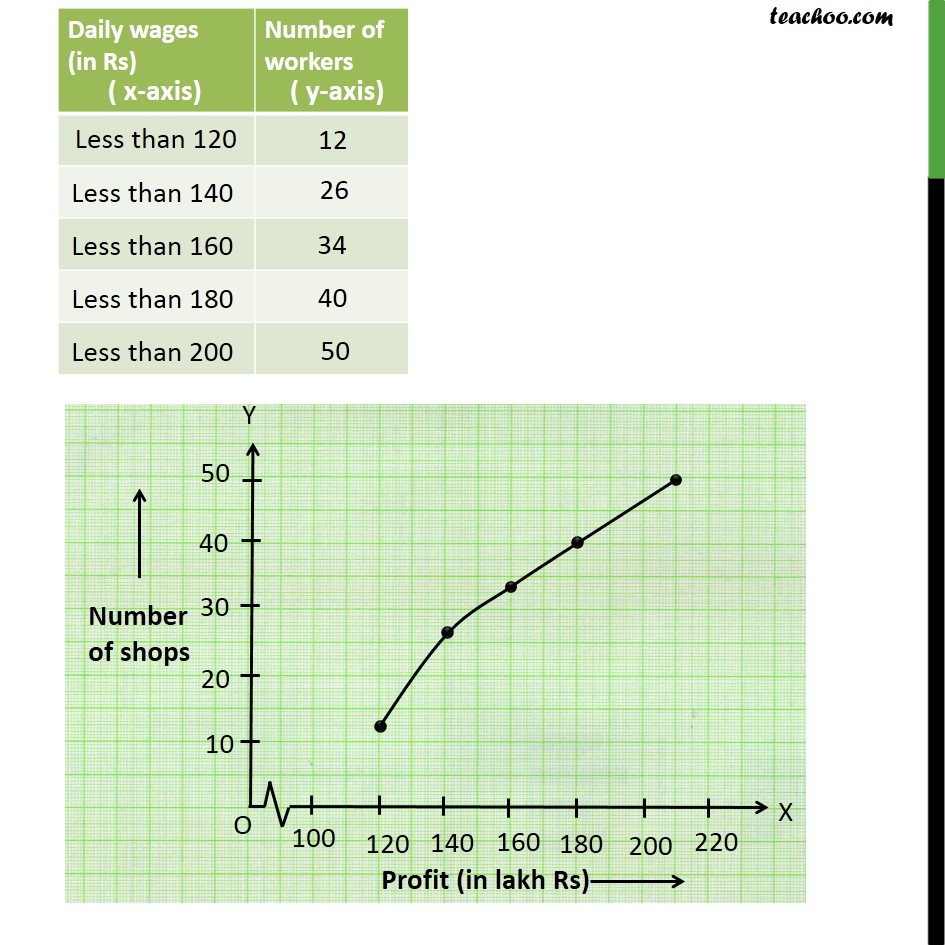1. Class 10
2. Important Questions for Exam - Class 10
3. Chapter 14 Class 10 Statistics

Transcript

Ex 14.4, 1 - Chapter 14 Class 10 The following distribution gives the daily income of 50 workers of a factory. Convert the distribution above to a less than type cumulative frequency distribution, and draw its ogive. Daily wages (in Rs) Number of workers Cumulative frequency 100 – 120 12 12 120 – 140 14 12+14=26 140 – 160 8 26+8=34 160 – 180 6 34+6=40 180 – 200 10 40+10=50 Daily wages Number of workers (in Rs) ( y-axis) ( x-axis) Less than 120 12 Less than 140 26 Less than 160 34 Less than 180 40 Less than 200 50 Now, drawing less than ogive

Chapter 14 Class 10 Statistics

Class 10
Important Questions for Exam - Class 10